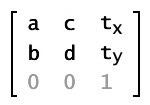# As3 Matrix 理解与应用## 一、介绍

Matrix 字面意思是矩阵， 是一个数学概念。这个词在本科线性代数里有详细说明，它是数的一个排列，支持好多种数学运算！在flash里，在3D运算中，矩阵 真的是无处不在。flash中，你要使一个可显示对象（DisplayObject ）旋转，倾斜，调位置你都可以通过矩阵来完成，虽然你还有其他更简单的方法，就是通过修改rotation, x, y 等值来达到同样的目的。在每个显示对象中，都有一个属性：transform. 这里就包含了显示对象的各种变换，其中一项，就是matrix！ 可见matrix在flash中也有重要的作用。

## 二、为什么是矩阵

### 三、矩阵

flash里 矩阵用于将点从一个坐标系转换到另一个坐标系， 使用下面的方程：## 四、应用

1. 获取对象里某一点在父对象中的位置。

```var p:Point = new Point( x0, y0 );
p = displayObject.transform.matrix.transformPoint( p );
```

2. 绘制渐变图

3. 中心旋转

```public function rotateAroundCenter (ob:DisplayObject, angleDegrees:Number):void
{
var matrix:Matrix = ob.transform.matrix;

var rect:Rectangle = ob.getBounds(ob.parent);

matrix.translate(- (rect.left + (rect.width/2)), - (rect.top + (rect.height/2)));

matrix.rotate((angleDegrees/180)*Math.PI);

matrix.translate(rect.left + (rect.width / 2), rect.top + (rect.height / 2));

ob.transform.matrix = matrix;
}
```

4. 绕点旋转

```// get matrix object from your MovieClip (mc)
var m:Matrix = mc.transform.matrix;

// set the point around which you want to rotate your MovieClip (relative to the MovieClip position)
var point:Point = new Point(10, 10);

// get the position of the MovieClip related to its origin and the point around which it needs to be rotated
point = m.transformPoint(point);
// set it
m.translate( -point.x, -point.y);

// rotate it of 30°
m.rotate(30 * (Math.PI / 180));

// and get back to its "normal" position
m.translate(point.x, point.y);

// finally, to set the MovieClip position, use this
mc.transform.matrix = m;

// or this
mc.x = m.tx;
mc.y = m.ty;
mc.rotation += 30;

```